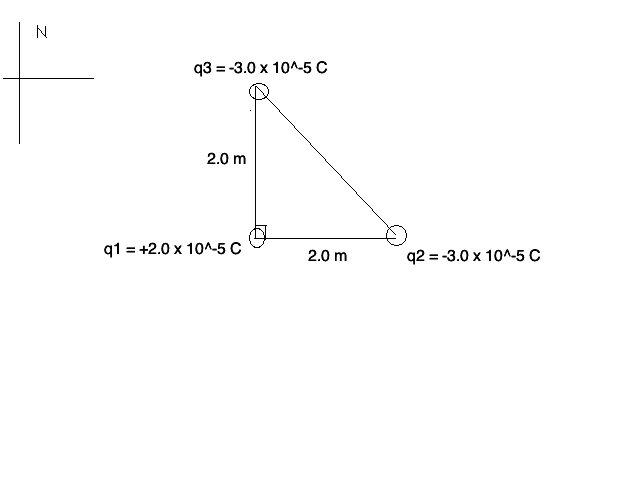# Coulomb's Law Problem

MiniOreo1998

## Homework Statement

Examine the charge distribution shown.a) Find the net force on charge 1
b) What is the net electric field acting on charge 1 (Haven't gotten to this yet. I know I won't be given the answer, however, even a hint on building intuition would be appreciated)

I've plugged my numbers into a very similar question that I've been given and have followed the procedure to the best of my ability. Feel free to tell me I'm completely wrong if that's the case.

## Homework Equations

F=kq1q2/r2
ε = kq1/r12 + kq2/r22

## The Attempt at a Solution

FE 2,1 = FE 3,1 = kq1q2/r2

FE 2,1 = FE 3,1 = (9.0x10^9)(2.0x10^-5)(3.0x10^-5)/(2.0)^2

FE 2,1 = FE 3,1 = 1.35 N

Fnet = √ 1.35^2+1.35^2 = 1.9N

Ɵ=tan-1 (1)

Ɵ=45 deg [NE]

Fnet = 1.9 N [45 deg NE]

Last edited:

## Answers and Replies

MiniOreo1998
Just realized there's a typo (new to the site, haven't found the "edit post" function yet, if there is one.) Anyway, Fnet = √ 1.35^2+1.25^2 = 3.6N is supposed to read Fnet = √ 1.35^2+1.35^2 = 3.6N (finger slipped)

EDIT: Found the edit button...Last edited:
Homework Helper
Hello MO, welcome to PF!

Lots more typos: relevant eqn misses factor 1/r2 (noticed there's a button for superscript and one for subscript too ?)

If you write
FE 2,1 = FE 3,1 = kq1q2/r2
it's unclear what you mean with the numbers: indices, exponents ?

Typo on the calculator too (either that, or the sqrt button is broken): ##\sqrt{1.35^2 +1.35^2} < 2 \times 1.35## ! Can never be so big as you found.

And for the potential you need a relevant equation too. It's not a vector but a scalar and you can add the separate contributions from q2 and q3

 sorry, misread potential instead of E fieldLast edited:
MiniOreo1998
Hello MO, welcome to PF!

Lots more typos: relevant eqn misses factor 1/r2 (noticed there's a button for superscript and one for subscript too ?)

If you write
FE 2,1 = FE 3,1 = kq1q2/r2
it's unclear what you mean with the numbers: indices, exponents ?

Typo on the calculator too (either that, or the sqrt button is broken): ##\sqrt{1.35^2 +1.35^2} < 2 \times 1.35## ! Can never be so big as you found.

And for the potential you need a relevant equation too. It's not a vector but a scalar and you can add the separate contributions from q2 and q3

I apologize, I've rarely typed out math and am just trying to get the hang of it. (for some reason I believed it would be understood, thanks for letting me know it comes off as nonsense)

I did mess up the relevant equation, (typed this out in a hurry, that's for sure... Will definitely slow down in the future)

As for FE 2, 1 and such, I was comparing electric forces of charge 2 (q2) to charge 1 (q1). Same with FE 3 being charge 3 (q3). Again, I can now see how that wouldn't make sense.

For the square root mishap; yes, I just realized I hadn't actually hit the square root button (it comes to 1.9091.... since I was given 2 sig digits, I'll go with 1.9 N instead)

Homework Helper
Don't worry too much, the intention is understood and correct. No need to apologize either, you, me, we all learn and that's what we're here for.

And writing FE 2->1 as FE 2,1 is understandable and does make sense.
1.9 N is what I found too. And NE idem. In an x, y coordinate system you could also say FE = (1.35, 1.35) N (where now the N stands for Newton; after all this is physics, not a navigation course, I suppose. But if the book says N, then it's obvious you have to say NE )

Now on to the potential. the electric field ##\vec E##

Last edited:
MiniOreo1998
As for potential:

εnet = (9.0 x 109)(3.0 x 10-5)/(2.0)2 + (9.0 x 109)(3.0 x 10-5)/(2.0)2 = 1.35 x 105 N [45 degrees to the right]

Last edited:
Homework Helper
 apologies, I read electric potential but the problem statement clearly says electric field.

What's the dimension of electric field strength ?
And how can the calculation 9 x 3 / 4 end up at 1.35 ?

Last edited:
MiniOreo1998
Net electric field:

ε = k q1 / r2 (measured in N/C, newtons per coulomb)

ε = (9.0 x 109) (2.0 x 10-5) / (2.0)2 = 95 000 N/C

Homework Helper
N/C is good.

But now you are calculating the electric field caused by q1 at a distance of 2 m. That doesn't give the net electric field acting on q1 at it's position in the origin.

MiniOreo1998
I'm picturing this in my head as q1 wanting to "act" with q2 and q3 equally. Since both q2 and q3 are negative and are both equal distances from q1, I'm assuming that q1 tries to "go toward" both of them (which in this case caused the 45 degree angle to the right as the direction). Is this getting any closer?

(9.0 x 109 (3.0 x 10-5) / 22 + (9.0 x 109 (3.0 x 10-5) / 22 = 135 000 N/C

k q2 / r2 + k q3 / r 2

I hate compass cards because they start in the wrong place (N instead of the x-axis), they run in the wrong direction (+ = clockwise instead of anti-clockwise) and they are too verbose (13 degrees North of West instead of 167 ##^\circ##). And degrees are also a source of misery. Mathematically (and therefore in physics too) the only right scale is ##2\pi## for a full circle. But what can you do